## Subnetting in Networking-

Before you go through this article, make sure that you have gone through the previous article on Subnetting.

We have discussed-

• Subnetting is a process of dividing a single network into multiple smaller networks.
• The number of sub networks created depends upon the requirement.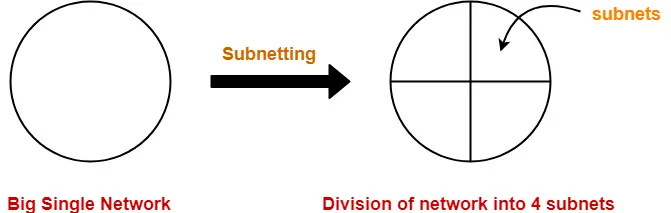## Arrangement Of Subnets-

• All the subnets are connected to an internal router.
• Internal router is connected to an external router.
• The link connecting the internal router with a subnet is called as an interface.

## Example-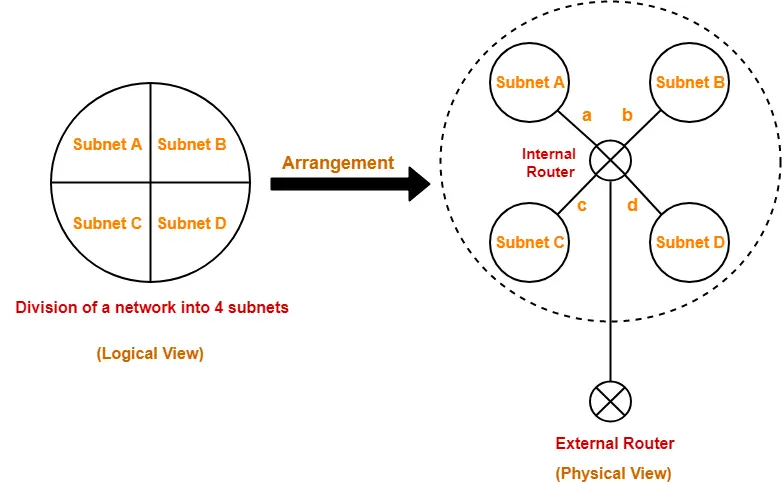## Working-

When a data packet arrives,

• External router forwards the data packet to the internal router.
• Internal router identifies the interface on which it should forward the incoming data packet.
• Internal router forwards the data packet on that interface.

## Routing Table-

• A table is maintained by the internal router called as Routing table.
• It helps the internal router to decide on which interface the data packet should be forwarded.

Routing table consists of the following three fields-

1. IP Address of the destination subnet
2. Subnet mask of the subnet
3. Interface

## Example-

Consider a network is subnetted into 4 subnets as shown in the above picture.

The IP Address of the 4 subnets are-

1. 200.1.2.0 (Subnet A)
2. 200.1.2.64 (Subnet B)
3. 200.1.2.128 (Subnet C)
4. 200.1.2.192 (Subnet D)

Then, Routing table maintained by the internal router looks like-

 Destination Subnet Mask Interface 200.1.2.0 255.255.255.192 a 200.1.2.64 255.255.255.192 b 200.1.2.128 255.255.255.192 c 200.1.2.192 255.255.255.192 d Default 0.0.0.0 e

Routing Table Example

When a data packet arrives to the internal router, it follows the following steps-

## Step-01:

Router performs the bitwise ANDing of-

• Destination IP Address mentioned on the data packet
• And all the subnet masks one by one.

## Step-02:

Router compares each result with their corresponding IP Address of the destination subnet in the routing table.

Then, following three cases may occur-

### Case-01:

If there occurs only one match,

• Router forwards the data packet on the corresponding interface.

### Case-02:

If there occurs more than one match,

• Router forwards the data packet on the interface corresponding to the longest subnet mask.

### Case-03:

If there occurs no match,

• Router forwards the data packet on the interface corresponding to the default entry.

## Note-01:

In fixed length subnetting,

• All the subnets have the same subnet mask.
• So, bitwise ANDing is performed only once.

If the result matches to any of the destination subnet IP Address,

• Router forwards the data packet on its corresponding interface.
• Otherwise, it is forwarded on the default interface.

## Note-02:

In variable length subnetting,

• All the subnets do not have the same subnet mask.
• So, bitwise ANDing is performed once with each subnet mask.
• Then, the above three cases are followed.

## Note-03:

• A host may also be directly connected to the router.
• In that case, there exists a host specific route from the router to the host.
• Router saves the IP Address of that host in the “Destination Network” column.
• Router saves 255.255.255.255 in the “Subnet Mask” column.
• The ANDing of its destination address and subnet mask yields the IP Address of the host.
• When a data packet arrives for that specific host, bitwise ANDing is performed.
• When the result of ANDing is the IP Address of the host, packet is forwarded to its host specific route.

## Note-04:

• Subnet mask for default route = 0.0.0.0
• Subnet mask for host specific route = 255.255.255.255

## Problem-01:

A router uses the following routing table-

 Destination Mask Interface 144.16.0.0 255.255.0.0 eth0 144.16.64.0 255.255.224.0 eth1 144.16.68.0 255.255.255.0 eth2 144.16.68.64 255.255.255.224 eth3

A packet bearing a destination address 144.16.68.117 arrives at the router. On which interface will it be forwarded?

1. eth0
2. eth1
3. eth2
4. eth3

## Solution-

Router performs the bitwise ANDing of-

• Destination address mentioned on the data packet
• And each subnet mask one by one.

### 1st Row-

144.16.68.117 AND 255.255.0.0

= 144.16.0.0

Since result is same as the given destination address, so a match occurs.

### 2nd Row-

144.16.68.117 AND 255.255.224.0

= 144.16.64.0

Since result is same as the given destination address, so a match occurs.

### 3rd Row-

144.16.68.117 AND 255.255.255.0

= 144.16.68.0

Since result is same as the given destination address, so a match occurs.

### 4th Row-

144.16.68.117 AND 255.255.255.224

= 144.16.68.96

Since result is not same as the given destination address, so a match does not occur.

Now,

• Clearly, there occurs more than one match.
• So, router forwards the packet on the interface corresponding to the longest subnet mask.
• Out of all, 255.255.255.0 is the longest subnet mask since it has maximum number of 1s.

So,

• Router forwards the packet on the interface corresponding to the subnet mask 255.255.255.0.
• The corresponding interface is eth2.

Thus, Option (C) is correct.

## Problem-02:

The routing table of a router is shown below-

 Destination Mask Interface 128.75.43.0 255.255.255.0 eth0 128.75.43.0 255.255.255.128 eth1 192.12.17.5 255.255.255.255 eth3 default eth2

On which interfaces will the router forward packets addressed to destination 128.75.43.16 and 192.12.17.10 respectively?

1. eth1 and eth2
2. eth0 and eth2
3. eth0 and eth3
4. eth1 and eth3

## Solution-

Router performs the bitwise ANDing of-

• Destination address mentioned on the data packet
• And each subnet mask one by one.

### 1st Row-

128.75.43.16 AND 255.255.255.0

= 128.75.43.0

Since result is same as the given destination address, so a match occurs.

### 2nd Row-

128.75.43.16 AND 255.255.255.128

= 128.75.43.0

Since result is same as the given destination address, so a match occurs.

### 3rd Row-

128.75.43.16 AND 255.255.255.255

= 128.75.43.16

Since result is not same as the given destination address, so a match does not occur.

Now,

• Clearly, there occurs more than one match.
• So, router forwards the packet on the interface corresponding to the longest subnet mask.
• Out of all, 255.255.255.128 is the longest subnet mask since it has maximum number of 1s.

So,

• Router forwards the packet on the interface corresponding to the subnet mask 255.255.255.128.
• The corresponding interface is eth1.

### 1st Row-

192.12.17.10 AND 255.255.255.0

= 192.12.17.0

Since result is not same as the given destination address, so a match does not occur.

### 2nd Row-

192.12.17.10 AND 255.255.255.128

= 192.12.17.0

Since result is not same as the given destination address, so a match does not occur.

### 3rd Row-

192.12.17.10 AND 255.255.255.255

= 192.12.17.10

Since result is not same as the given destination address, so a match does not occur.

Now,

• Clearly, there occurs no match.
• So, router forwards the packet on the interface corresponding to the default entry.
• The corresponding interface is eth2.

Thus, Option (A) is correct.

## Problem-03:

Host specific route has a subnet mask of _____ in the routing table.

1. 255.255.255.255
2. 0.0.0.0
3. 255.0.0.0
4. 0.0.0.255

## Solution-

Option (A) is correct.

## Problem-04:

Default route has a subnet mask of _____ in the routing table.

1. 255.255.255.255
2. 0.0.0.0
3. 255.0.0.0
4. 0.0.0.255

## Solution-

Option (B) is correct.

## Problem-05:

Default route can be described as-

1. Destination values of 0.0.0.0 in the routing table
2. It can be used if network has only one next hop router
3. It is useful in keeping routing table small
4. All of the above

## Solution-

Option (D) is correct.

To gain better understanding about Routing Table,

Watch this Video Lecture

Next Article- Use of Subnet Mask

Get more notes and other study material of Computer Networks.

Watch video lectures by visiting our YouTube channel LearnVidFun.

## Subnetting in Networking-

In networking,

• The process of dividing a single network into multiple sub networks is called as subnetting.
• The sub networks so created are called as subnets.

## Example-

Following diagram shows the subnetting of a big single network into 4 smaller subnets-The two main advantages of subnetting a network are-

• It improves the security.
• The maintenance and administration of subnets is easy.

## Subnet ID-

• Each subnet has its unique network address known as its Subnet ID.
• The subnet ID is created by borrowing some bits from the Host ID part of the IP Address.
• The number of bits borrowed depends on the number of subnets created.

## Types of Subnetting-

Subnetting of a network may be carried out in the following two ways-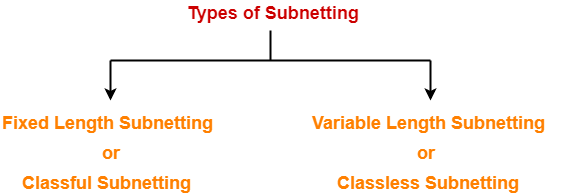1. Fixed Length Subnetting
2. Variable Length Subnetting

## 1. Fixed Length Subnetting-

Fixed length subnetting also called as classful subnetting divides the network into subnets where-

• All the subnets are of same size.
• All the subnets have equal number of hosts.
• All the subnets have same subnet mask.

## 2. Variable Length Subnetting-

Variable length subnetting also called as classless subnetting divides the network into subnets where-

• All the subnets are not of same size.
• All the subnets do not have equal number of hosts.
• All the subnets do not have same subnet mask.

## Subnetting Examples-

Now, we shall discuss some examples of subnetting a network-

## Example-01:

Consider-

• We have a big single network having IP Address 200.1.2.0.
• We want to do subnetting and divide this network into 2 subnets.

Clearly, the given network belongs to class C.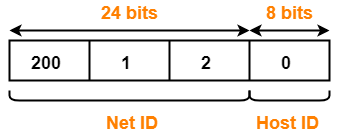For creating two subnets and to represent their subnet IDs, we require 1 bit.

So,

• We borrow one bit from the Host ID part.
• After borrowing one bit, Host ID part remains with only 7 bits.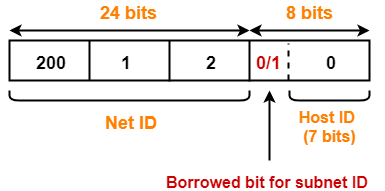• If borrowed bit = 0, then it represents the first subnet.
• If borrowed bit = 1, then it represents the second subnet.

IP Address of the two subnets are-

• 200.1.2.00000000 = 200.1.2.0
• 200.1.2.10000000 = 200.1.2.128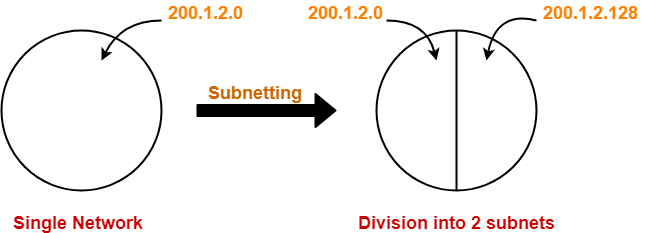## For 1st Subnet-

• IP Address of the subnet = 200.1.2.0
• Total number of IP Addresses = 27 = 128
• Total number of hosts that can be configured = 128 – 2 = 126
• Range of IP Addresses = [200.1.2.00000000, 200.1.2.01111111] = [200.1.2.0, 200.1.2.127]

## For 2nd Subnet-

• IP Address of the subnet = 200.1.2.128
• Total number of IP Addresses = 27 = 128
• Total number of hosts that can be configured = 128 – 2 = 126
• Range of IP Addresses = [200.1.2.10000000, 200.1.2.11111111] = [200.1.2.128, 200.1.2.255]

## Example-02:

Consider-

• We have a big single network having IP Address 200.1.2.0.
• We want to do subnetting and divide this network into 4 subnets.

Clearly, the given network belongs to class C.For creating four subnets and to represent their subnet IDs, we require 2 bits.

So,

• We borrow two bits from the Host ID part.
• After borrowing two bits, Host ID part remains with only 6 bits.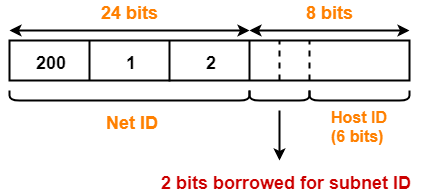• If borrowed bits = 00, then it represents the 1st subnet.
• If borrowed bits = 01, then it represents the 2nd subnet.
• If borrowed bits = 10, then it represents the 3rd subnet.
• If borrowed bits = 11, then it represents the 4th subnet.

IP Address of the four subnets are-

• 200.1.2.00000000 = 200.1.2.0
• 200.1.2.01000000 = 200.1.2.64
• 200.1.2.10000000 = 200.1.2.128
• 200.1.2.11000000 = 200.1.2.192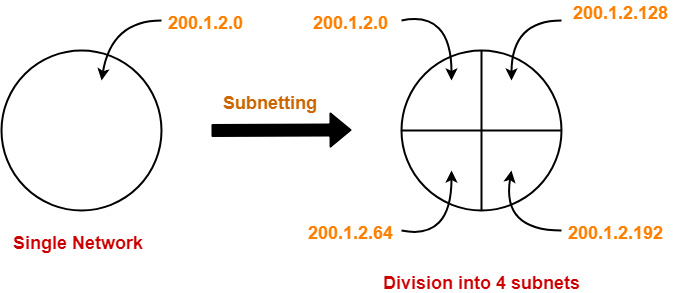## For 1st Subnet-

• IP Address of the subnet = 200.1.2.0
• Total number of IP Addresses = 26 = 64
• Total number of hosts that can be configured = 64 – 2 = 62
• Range of IP Addresses = [200.1.2.00000000, 200.1.2.00111111] = [200.1.2.0, 200.1.2.63]

## For 2nd Subnet-

• IP Address of the subnet = 200.1.2.64
• Total number of IP Addresses = 26 = 64
• Total number of hosts that can be configured = 64 – 2 = 62
• Range of IP Addresses = [200.1.2.01000000, 200.1.2.01111111] = [200.1.2.64, 200.1.2.127]

## For 3rd Subnet-

• IP Address of the subnet = 200.1.2.128
• Total number of IP Addresses = 26 = 64
• Total number of hosts that can be configured = 64 – 2 = 62
• Range of IP Addresses = [200.1.2.10000000, 200.1.2.10111111] = [200.1.2.128, 200.1.2.191]

## For 4th Subnet-

• IP Address of the subnet = 200.1.2.192
• Total number of IP Addresses = 26 = 64
• Total number of hosts that can be configured = 64 – 2 = 62
• Range of IP Addresses = [200.1.2.11000000, 200.1.2.11111111] = [200.1.2.192, 200.1.2.255]

## Example-03:

Consider-

• We have a big single network having IP Address 200.1.2.0.
• We want to do subnetting and divide this network into 3 subnets.

Here, the subnetting will be performed in two steps-

1. Dividing the given network into 2 subnets
2. Dividing one of the subnets further into 2 subnets

## Step-01: Dividing Given Network into 2 Subnets-

The subnetting will be performed exactly in the same way as performed in Example-01.

After subnetting, we have-## Step-02: Dividing One Subnet into 2 Subnets-

• We perform the subnetting of one of the subnets further into 2 subnets.
• Consider we want to do subnetting of the 2nd subnet having IP Address 200.1.2.128.

For creating two subnets and to represent their subnet IDs, we require 1 bit.

So,

• We borrow one more bit from the Host ID part.
• After borrowing one bit, Host ID part remains with only 6 bits.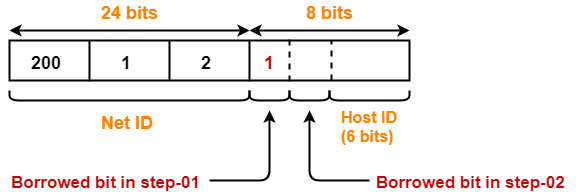• If 2nd borrowed bit = 0, then it represents one subnet.
• If 2nd borrowed bit = 1, then it represents the other subnet.

IP Address of the two subnets are-

• 200.1.2.10000000 = 200.1.2.128
• 200.1.2.11000000 = 200.1.2.192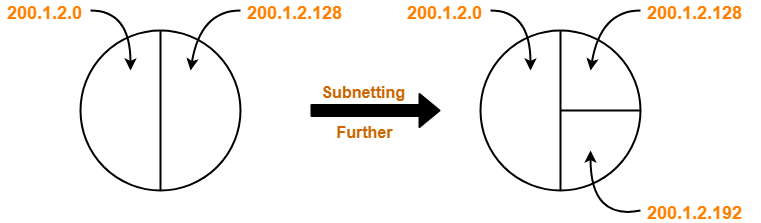Finally, the given single network is divided into 3 subnets having IP Address-

• 200.1.2.0
• 200.1.2.128
• 200.1.2.192

## For 1st Subnet-

• IP Address of the subnet = 200.1.2.0
• Total number of IP Addresses = 27 = 128
• Total number of hosts that can be configured = 128 – 2 = 126
• Range of IP Addresses = [200.1.2.00000000, 200.1.2.01111111] = [200.1.2.0, 200.1.2.127]

## For 2nd Subnet-

• IP Address of the subnet = 200.1.2.128
• Total number of IP Addresses = 26 = 64
• Total number of hosts that can be configured = 64 – 2 = 62
• Range of IP Addresses = [200.1.2.10000000, 200.1.2.10111111] = [200.1.2.128, 200.1.2.191]

## For 3rd Subnet-

• IP Address of the subnet = 200.1.2.192
• Total number of IP Addresses = 26 = 64
• Total number of hosts that can be configured = 64 – 2 = 62
• Range of IP Addresses = [200.1.2.11000000, 200.1.2.11111111] = [200.1.2.192, 200.1.2.255]

## Point-01:

During subnetting,

• We have to face a loss of IP Addresses.
• This is because two IP Addresses are wasted for each subnet.

## Point-02:

 Subnetting leads to complicated communication process.

After subnetting, the communication process becomes complex involving the following 4 steps-

1. Identifying the network
2. Identifying the sub network
3. Identifying the host
4. Identifying the process

## Problem-01:

Suppose a network with IP Address 192.16.0.0. is divided into 2 subnets, find number of hosts per subnet.

Also for the first subnet, find-

2. First Host ID
3. Last Host ID

## Solution-

• Given IP Address belongs to class C.
• So, 24 bits are reserved for the Net ID.
• The given network is divided into 2 subnets.
• So, 1 bit is borrowed from the host ID part for the subnet IDs.
• Then, Number of bits remaining for the Host ID = 7.
• Thus, Number of hosts per subnet = 27 = 128.

### For 1st Subnet-

• First Host ID = 192.16.0.00000001 = 192.16.0.1
• Last Host ID = 192.16.0.01111110 = 192.16.0.126

## Problem-02:

What is not true about subnetting?

1. It is applied for a single network
2. It is used to improve security
3. Bits are borrowed from network portion
4. Bits are borrowed from Host portion

## Solution-

Clearly, Option (C) is correct.

## Problem-03:

In a class B, network on the internet has a subnet mask of 255.255.240.0. What is the maximum number of hosts per subnet?

1. 4096
2. 4094
3. 4092
4. 4090

## Solution-

• Number of bits reserved for network ID in the given subnet mask = 20.
• So, Number of bits reserved for Host ID = 32 – 20 = 12 bits.
• Thus, Number of hosts per subnet = 212 – 2 = 4094.
• In class B, 16 bits are reserved for the network.
• So, Number of bits reserved for subnet ID = 20 – 16 = 4 bits.
• Number of subnets possible = 24 = 16.
• Thus, Option (B) is correct.

To gain better understanding about IPv4 Subnetting,

Watch this Video Lecture